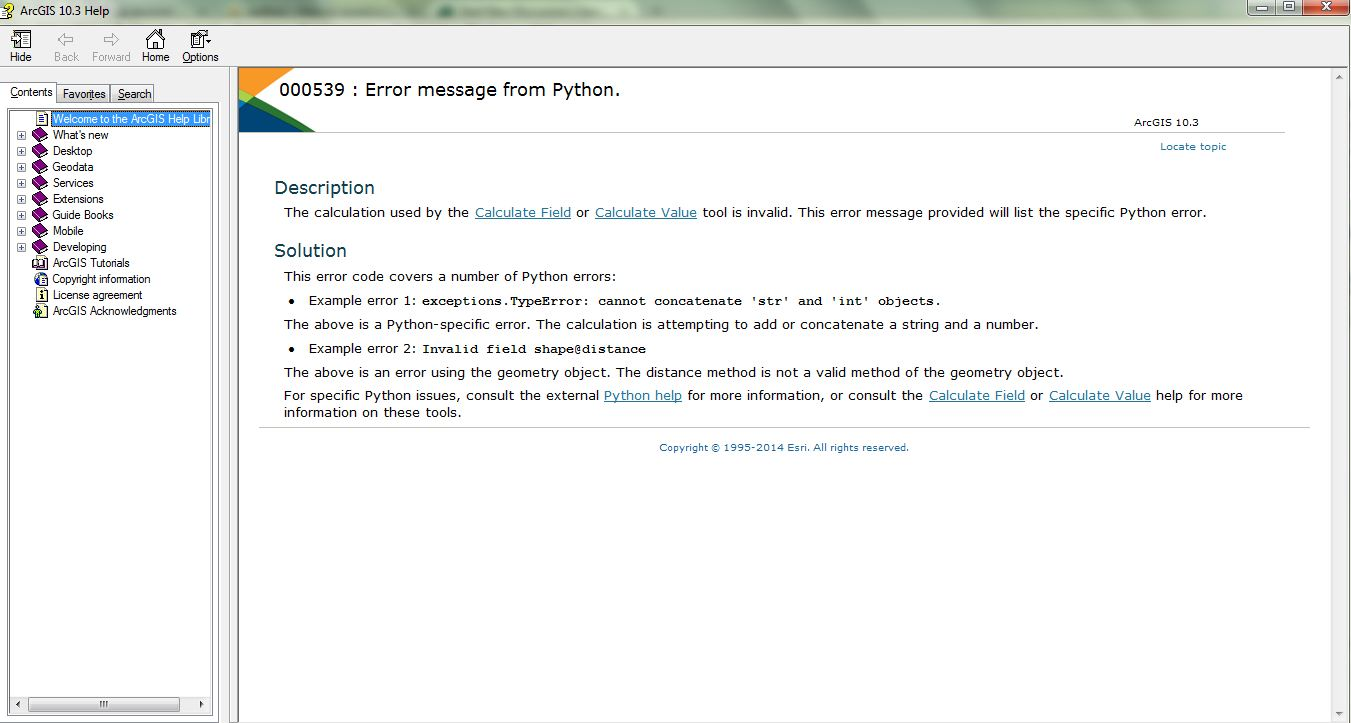# Round values in an attribute table to the nearest nth value using the Field Calculator and a Python expression.

2305
4
03-01-2016 10:19 PMOccasional Contributor

Hello guys

I want to use the Field Calculator in ArcMap to round an existing column to two decimals. Currently I have a column (AREA_Ha) that is 3 decimal places long and would like to simply round it down to 2 decimals.I have tried this ( `round(!AREA_Ha!, 2))` but get this error message " There was a failure during processing, check the Geoprocessing Results window for details`.`Tags (3)
1 Solution

Accepted SolutionsbyMVP Esteemed Contributor

str(round(float(a.split(" ")),2) ) + " ha"

but it is getting ridiculously convoluted, the area calculation should have been sent to a numeric field, rounding there, and then, converted to string in a subsequent field

4 RepliesbyMVP Esteemed Contributor

You can tell because it is left justified and contains 'ha' at the end. So however you created the area field wasn't a true area field but the representation of an area field.

The most descriptive is to follow

a = '1.2345 ha'

round(float(a.split(" ")),2)

yields 1.23

where 'a' is your field nameOccasional Contributor

Thanks Dan but is to possible to add the unit abbreviation eg.1.23 HabyMVP Esteemed Contributor

str(round(float(a.split(" ")),2) ) + " ha"

but it is getting ridiculously convoluted, the area calculation should have been sent to a numeric field, rounding there, and then, converted to string in a subsequent fieldOccasional Contributor